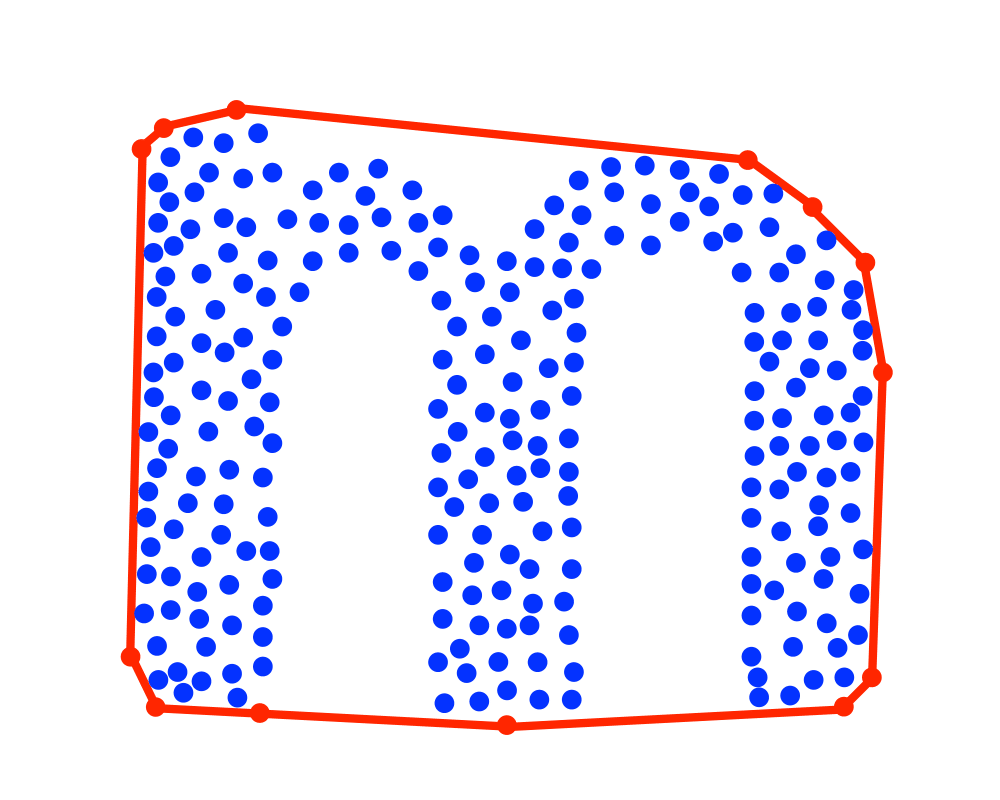# Convex HullInputOutput

Input Description: A set $$S$$ of $$n$$ points in $$d$$-dimensional space.
Problem: Find the smallest convex polygon containing all the points of $$S$$.

Excerpt from The Algorithm Design Manual: Finding the convex hull of a set of points is the most elementary interesting problem in computational geometry, just as minimum spanning tree is the most elementary interesting problem in graph algorithms. It arises because the hull quickly captures a rough idea of the shape or extent of a data set.

Convex hull also serves as a first preprocessing step to many, if not most, geometric algorithms. For example, consider the problem of finding the diameter of a set of points, which is the pair of points a maximum distance apart. The diameter will always be the distance between two points on the convex hull. The O(n \lg n). algorithm for computing diameter proceeds by first constructing the convex hull, then for each hull vertex finding which other hull vertex is farthest away from it. This so-called rotating-calipers'' method can be used to move efficiently from one hull vertex to another.

Go To Main Page